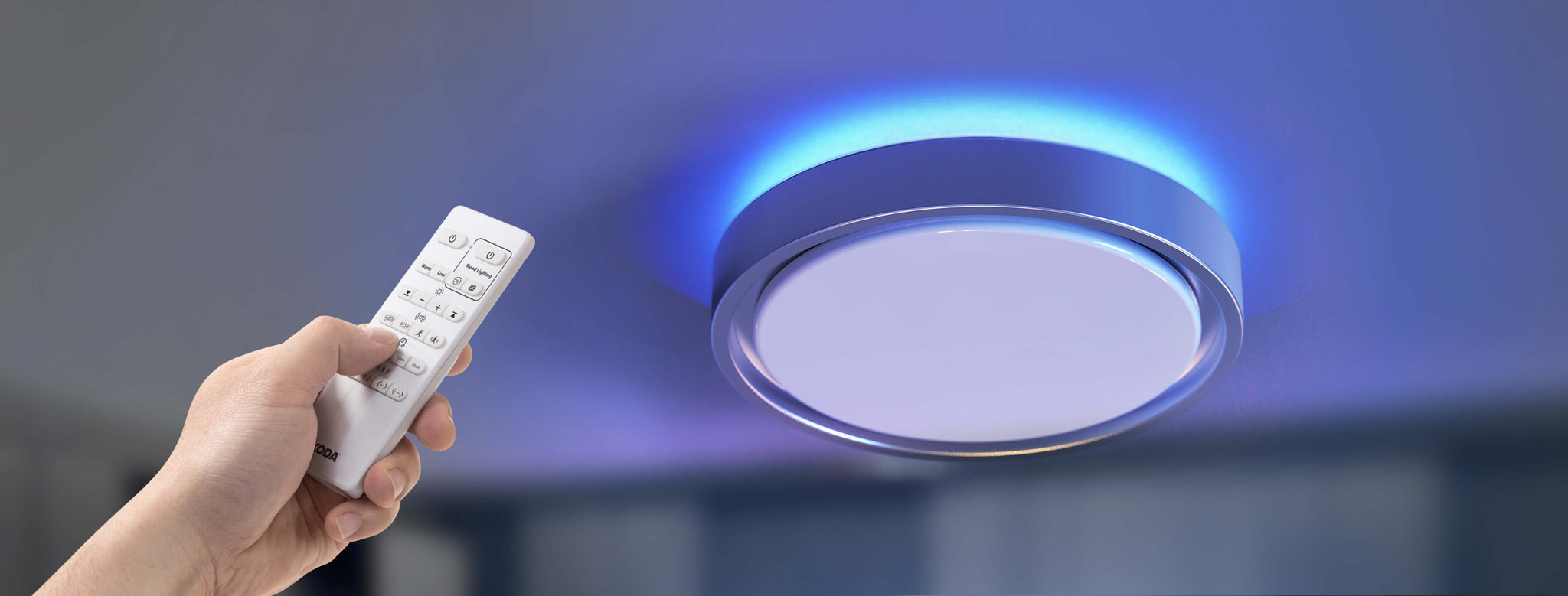Home / News, Events and Blog / What are Watts? Energy Consumption Explained# What are Watts? Energy Consumption Explained

## Watt and Energy Consumption

When it comes to predicting how your lights and other appliances will impact your power bill, it's the rate of consumption that matters most. That measurement is the wattage, but the relationship between the stated wattage of a bulb and the monthly energy consumption it reflects is more complicated because the wattage of the bulb reflects the amount of power consumed per second. That's what watts units are, 40 joules of power consumed in a second. So, for example, a 60W bulb would be 2,400 joules per second of power consumed. Your power company doesn't measure energy costs in those terms, though. They use kilowatt hours.

## Relationship Between Wattage and Energy Consumption

So if watts are the energy consumed in a second, then how do they relate to the energy units you see on a power bill? It's simple. If a single watt is a statement of energy consumed in a second, then to calculate the bill, power companies calculate the number of watts consumed by your entire home over the course of an hour. Since the average customer uses more than 1,000 watts per hour and the cost of that much energy is typically very low, it is usually the basic unit of measurement on a power bill. That's why your LED ceiling fixtures, freezers, and other Energy Star compliant appliances list their annual and monthly consumption numbers in kWh.

##Energy and its Units

So to answer the question, do watts determine energy consumption? Turns out to be yes, but they're such a small unit of measurement that we need to consolidate them into kilowatts consumed per hour. Typically, this is calculated by looking at the amount of electric force delivered and the rate of delivery. That's why there are three important numbers to understand about energy consumption.

• Amperes measure the amount of force delivered
• Volts measure the rate of delivery
• Watts are derived by calculating the amount of force delivered and rate to get power consumed, so W = A*V

Many appliances are high voltage but low amperage or vice versa, which is why they are constructed with built-in power inverters that can change the flow of electricity from the home's standard to the appliance's needed voltage.

## How Many Watts are in LED Lights?

This question is not one with a single answer, because LED light fixtures come with a wide variety of power outputs. It depends a lot on whether you're talking about a single bulb or a large collection of smaller ones, as well as what light intensity the light provides. When compared to halogen, incandescent, and fluorescent bulbs, LEDs consume much less power, though. Buy energy star efficient products to ensure you are saving the most money and energy.